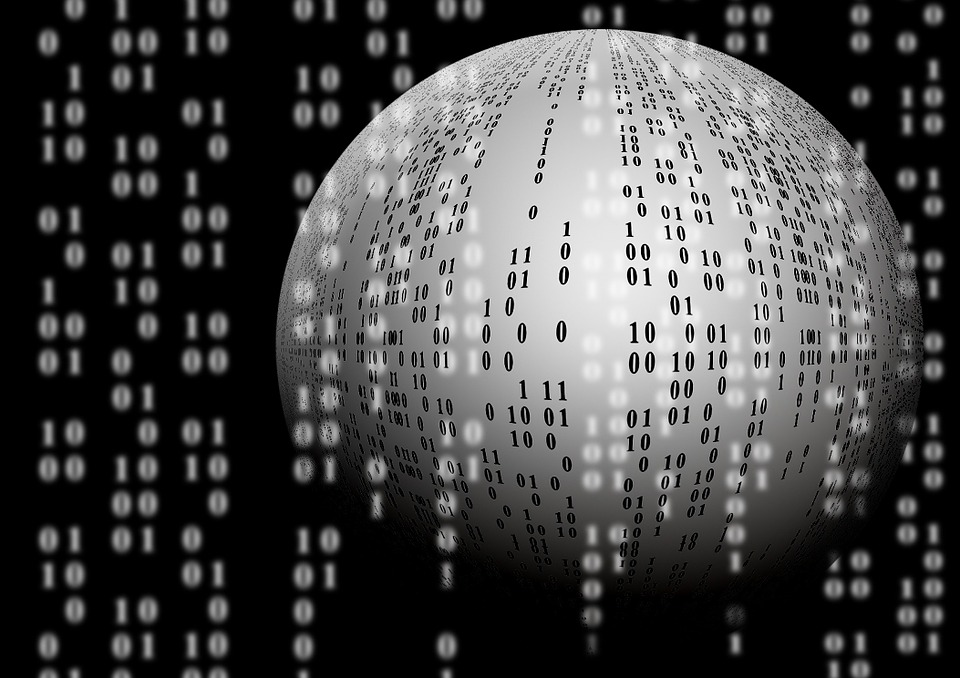# Exploring the Potential and Challenges of Quantum Computing

Quantum computing is a new paradigm in computing that leverages the principles of quantum mechanics to perform calculations that are not feasible with classical computers. Quantum mechanics is a branch of physics that describes the behavior of matter and energy on a microscopic scale, and it allows for phenomena that cannot be explained by classical physics. In a classical computer, information is stored in binary digits or bits that can have a value of either 0 or 1. In contrast, in a quantum computer, information is stored in quantum bits or qubits that can be in a superposition of both 0 and 1 state simultaneously, allowing for the potential of exponentially faster computations.

The basic unit of a quantum computer is the qubit, which is the quantum analogue of a classical bit. A qubit can be realized in many ways, but one of the most common approaches is to use the spin of a particle, such as an electron or a proton. The spin of a particle can be either up or down, corresponding to the states 0 and 1 in a classical bit. However, in a quantum computer, a qubit can be in a superposition of both up and down states simultaneously, meaning that it can represent both 0 and 1 at the same time.

This property of superposition is what gives quantum computers their power. While a classical computer can only perform one calculation at a time, a quantum computer can perform many calculations simultaneously by putting all of the possible outcomes of a calculation into a superposition. For example, a quantum computer with 100 qubits can perform 2^100 calculations at once, which is a staggering number that cannot be matched by classical computers.

Another property of quantum mechanics that is important for quantum computing is entanglement. Entanglement occurs when two or more qubits are linked in such a way that the state of one qubit is dependent on the state of the others. This means that if one qubit is measured, the state of the other qubits can be immediately determined, even if they are far apart from each other. This property allows for the creation of quantum algorithms that can solve problems that are not feasible with classical computers.Exploring the Potential and Challenges of Quantum Computing

One of the most famous quantum algorithms is Shor's algorithm, which is used for factoring large numbers. Factoring large numbers is a problem that is believed to be hard for classical computers, but Shor's algorithm can solve it in polynomial time on a quantum computer. This algorithm has important implications for cryptography because many encryption methods rely on the difficulty of factoring large numbers.

Another important quantum algorithm is Grover's algorithm, which is used for searching unstructured databases. Grover's algorithm can search a database of N items in O(sqrt(N)) time, which is faster than the O(N) time required by classical algorithms

Despite their potential advantages, quantum computers are still in their early stages of development and have many challenges to overcome before they can be used for practical applications. One of the biggest challenges is maintaining the fragile quantum state of qubits, which can be easily disrupted by environmental factors such as temperature and electromagnetic radiation. This problem is addressed by using techniques such as error correction, which involves redundantly encoding information to protect against errors.

In addition to the challenges of building and maintaining qubits, another challenge is developing algorithms that can take advantage of the potential speedup of quantum computers. Not all problems can be solved faster on a quantum computer than on a classical computer, and finding the right problems to solve with quantum computers is an active area of research.

Despite these challenges, quantum computing has the potential to revolutionize many fields, including cryptography, materials science, and drug discovery. As research in this field continues, we can expect to see exciting developments and advancements in both the theory and practical applications of quantum computing.